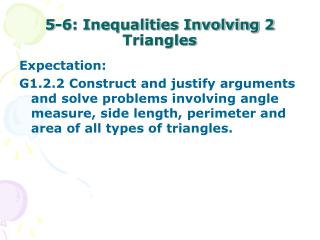DownloadDownload Presentation5-6: Inequalities Involving 2 Triangles

# 5-6: Inequalities Involving 2 Triangles

Download Presentation## 5-6: Inequalities Involving 2 Triangles

- - - - - - - - - - - - - - - - - - - - - - - - - - - E N D - - - - - - - - - - - - - - - - - - - - - - - - - - -
##### Presentation Transcript

1. 5-6: Inequalities Involving 2 Triangles • Expectation: • G1.2.2 Construct and justify arguments and solve problems involving angle measure, side length, perimeter and area of all types of triangles.

2. In the triangles below, 2 pairs of corresponding sides are congruent. Compare the third sides. x 2.17 2.17 y 82° 32° 2.67 2.67

3. SAS Inequality (Hinge Theorem) • If two sides of one triangle are congruent to 2 sides of a second triangle, but the included angle of the first is greater than the included angle of the second, then the 3rd side of the first is _______________ than the 3rd side of the second.

4. Two pairs of corresponding sides are congruent in the triangles below. Compare the included angles for the given congruent sides.

5. SSS Inequality • If two sides of one triangle are congruent to 2 sides of a second triangle, but the third side of the first is longer than the third side of the second, then the included angle between the pair of congruent sides of the first is ______________ than the included angle between the pair of congruent sides of the second.

6. Use the figure to compare the indicated measures. E 8 D 10 10 9 6 C 8 A B 9 a. m∠ADB and m∠DBC

7. Use the figure to compare the indicated measures. E 8 D 10 10 9 6 C 8 A B 9 b. m∠EAD and m∠BCD

8. In ΔABC and ΔDEF below (not drawn to scale) AB, BC, DE and EF are all 12 units long. If m∠A = 30° and m∠F = 50°, compare AC and DF. B E • AC = DF • AC > DF • AC < DF • not enough information given to determine an answer. A D C F

9. Given : QC bisects PD and m∠PCQ > m∠DCQ Prove: m∠D > m∠P P C D Q

10. Assignment: pages 277, # 15 - 29 (odds)